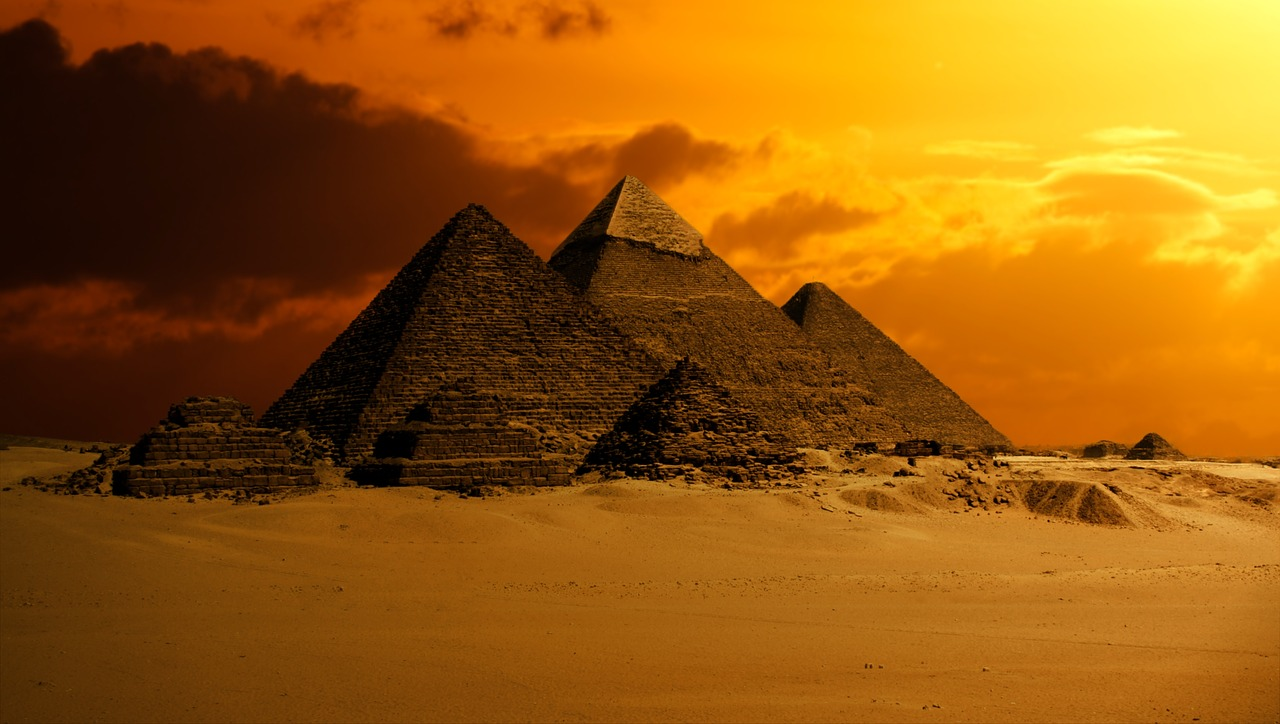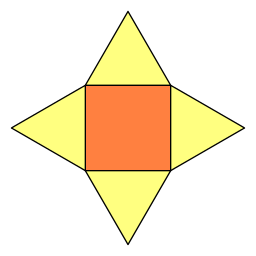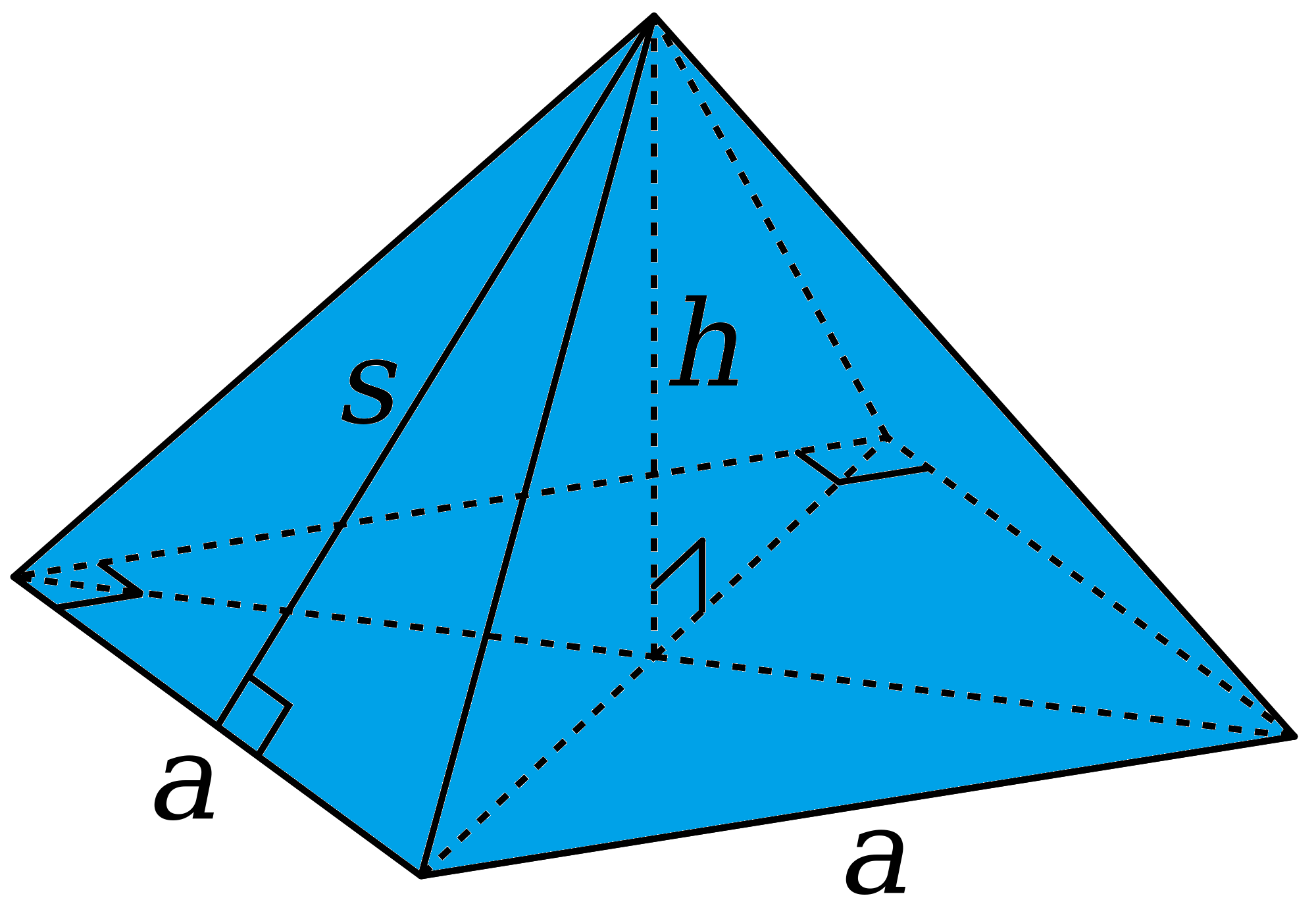# Square PyramidA square pyramid is a familiar-looking polyhedron (flat-sided three-dimensional figure), which has a square as its base, and four faces, shaped like triangles, that meet at a point, called the apex.

The Ancient Egyptian pyramids were (essentially) square pyramids (they were remarkably accurate for their time, but they were a bit off being square).

Square pyramids have five faces, five vertices and eight edges.## Building a Square Pyramid

You can make a square pyramid yourself by using a template like the following net (template), cutting it out along the outside lines, folding it along the inside lines and taping it together along the edges.## Finding the Surface Area and Volume of a Square Pyramid

### Finding the Surface Area of a Square Pyramid

Because the base of a square pyramid is, surprisingly enough, a square, we can calculate its area easily. It's just the square of the side-length of the base. The rest of the surface area depends on whether the pyramid is a right square pyramid (apex is directly over the centre of the base) or not.

For a right square pyramid, the four triangular faces will be equal, so we can calculate the surface area as follows:We can split the surface area of a square pyramid up into two parts:

1. The Area of the Base ($=a^2$ in the picture\).
2. The Area of the Slanted Sides

When the slanted sides are all the same, the surface area of the square pyramid is given by the formula:

$\text{Surface Area} = (\text{Base Area}) + \dfrac{1}{2} (\text{4a})(\text{Slant Height}) = a^2 + 2a (\text{Slant Height}).$
The slant height is labelled $s$ in the picture on the left.

For example, the surface area of a square pyramid with base side-length $10$ m, and slant height $5$ m is

\begin{align*} \text{Surface Area}& = a^2 + 2a (\text{Slant Height})\\ &= 10^2 + 2(10)(5)\\ &= 100 + 100\\ &= 200 \text{ m}^2. \end{align*}

If the pyramid is oblique, you can still find the surface area. You just have to calculate the areas of the slanted sides separately, and then find the total of the areas of the slanted sides and the base.

### Finding the Volume of a Square Pyramid

Like any pyramid, the volume of a square pyramid is equal to $\dfrac{1}{3}$ of the volume of the rectangular prism with the same height and (square) base. So,

$\text{Volume} = \dfrac{1}{3} (\text{Base Area})(\text{Height}) = \dfrac{1}{3}\;a^2 (\text{Height}).$

For example, the volume of a pyramid with base side-length $10$ m and height $6$ m is

\begin{align*} \text{Volume}& = \dfrac{1}{3}\;a^2 (\text{Height})\\ &= \dfrac{1}{3}\;10^2 (6)\\ &= \dfrac{1}{3}(100)(6)\\ &= 200 \text{ m}^3. \end{align*}

### Description

There are several lessons related to 3D geometry such as

1. Euler's formula
2. Vertices, Edges and Faces
3. Volumes of 3D shapes
4. etc

Even though we've titled this lesson series to be more inclined for Year 10 or higher students, however, these lessons can be read and utilized by lower grades students.

### Prerequisites

Understanding of 3D shapes

### Audience

Year 10 or higher, but suitable for Year 8+ students

### Learning Objectives

Get to know 3D Geometry

Author: Subject Coach
You must be logged in as Student to ask a Question.